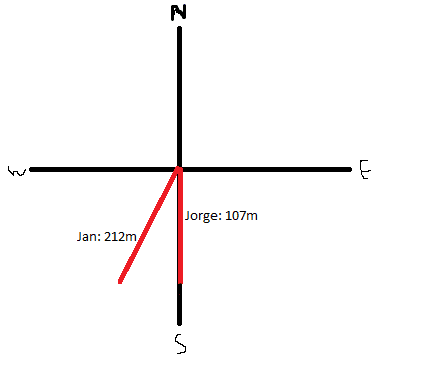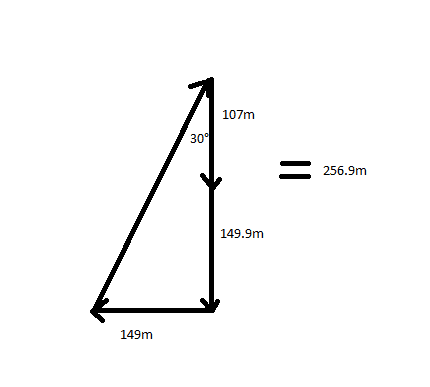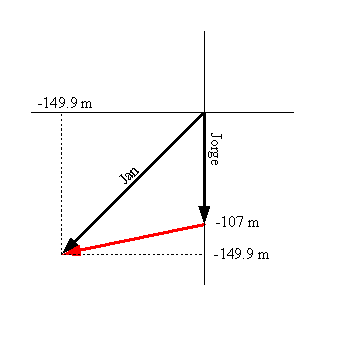# Running and Location/Distance Question

• physicsnobrain
Why not? If Jorge wanted to walk from where he is to where Jan is, he could either follow the red line in a straight line, or alternatively, he might take...Why not? If Jorge wanted to walk from where he is to where Jan is, he could either follow the red line in a straight line, or alternatively, he might take the path that goes through the center of the two circles.

## Homework Statement

Jan and Jorge ran away from a starting point. If Jorge ran 107m South and Jan ran 212m to the southwest, where is Jan relative to Jorge?

## Homework Equations

sin theta = opp/hyp

## The Attempt at a Solution

Jorge runs directly south and Jan runs southwest. So this forms a right triangle right? From there I solve for theta angle, which is located at their starting point. I solve it to be about 60 degrees. Next I solve for the opposite side as this is their separation in meters, correct? I solve this to be 183.597 meters.

My final answer: relative to jorge, jan is 184m directly west.

Did I solve this correctly?

I don't know. But in problems like this, a picture helps greatly.

OK, I just drew this. This is how I envision the question to be:Does this help illustrate the question?

Anyone have any thoughts?

Maybe its a vector addition question?

physicsnobrain said:
Maybe its a vector addition question?

Close. If Jorge at his new location were to turn and point towards Jan at her new location, what would be the distance to Jan and the direction? You'll want to treat their displacements as vectors (or at least deal with them component-wise to do your calculations).

gneill said:
Close. If Jorge at his new location were to turn and point towards Jan at her new location, what would be the distance to Jan and the direction? You'll want to treat their displacements as vectors (or at least deal with them component-wise to do your calculations).

Ok. Well in this case I will have to split up the two runners into their x and y components. Jorge is straighforward, however Jan has an angle. Now my question is, is the angle of southwest 225 degrees?

In this case, if it is 225 degrees I will be able to solve the question.

physicsnobrain said:
Ok. Well in this case I will have to split up the two runners into their x and y components. Jorge is straighforward, however Jan has an angle. Now my question is, is the angle of southwest 225 degrees?

In this case, if it is 225 degrees I will be able to solve the question.

Yup. That's the angle from the easterly direction (or positive X-axis if you prefer to think of it that way).

Ok, so after running the numbers I get that relative to jorge, jill is 297m away at 70 degrees south of west.

I got this by adding up the vectors. Then I created a right triangle, solved for the hypotenus, which was there distance between each other, then solved the angle and did 90 - theta to find the angle below horizontal.

Are my numbers correct?

physicsnobrain said:
Ok, so after running the numbers I get that relative to jorge, jill is 297m away at 70 degrees south of west.

I got this by adding up the vectors. Then I created a right triangle, solved for the hypotenus, which was there distance between each other, then solved the angle and did 90 - theta to find the angle below horizontal.

Are my numbers correct?

The numbers don't look right to me. What components did you get for Jorge's and Jill's locations? It's not clear to me what right angle triangle you constructed. Can you draw a picture?

Jacks x coordinate = 0, y coordinate = 107m south
Jill x coordinate = 212sin45 = 149.9m west, y coordinate = 212cos45 = 149.9m south

Here I add them up to get this right angle triangle:Okay, here's the thing. If you want to find the distance between two points, you need to take a difference, not add the two locations. On your diagram, the vector you want would be from the tip of Jorge's arrow to the tip of Jan's arrow (I've reverted to using the names given in the original problem description). So like this:The red vector is the one you want. It points from Jorge's current position to Jan's current position.

#### Attachments

gneill said:
Okay, here's the thing. If you want to find the distance between two points, you need to take a difference, not add the two locations. On your diagram, the vector you want would be from the tip of Jorge's arrow to the tip of Jan's arrow (I've reverted to using the names given in the original problem description). So like this:The red vector is the one you want. It points from Jorge's current position to Jan's current position.

ok so you're saying for the y direction it should be 149m - 107m. That does make sense.

physicsnobrain said:
ok so you're saying for the y direction it should be 149m - 107m. That does make sense.

Why not? If Jorge wanted to walk from where he is to where Jan is, he could either follow the red line in a straight line, or alternatively, he might take the scenic route going straight down from y = -107 to -149, then straight across from x = 0 to -149. Those two legs make up the components of the red vector.

running the numbers now I get relative to jorge, jan is about 155m away at 16 degrees S of W.

physicsnobrain said:
running the numbers now I get relative to jorge, jan is about 155m away at 16 degrees S of W.

The distance is about right (should be just a tad more -- watch out for rounding errors in intermediate steps. Keep a few extra digits in intermediate values). The angle is suspect; can you post your calculation?

I calculated first the angle by inverse tan(149m/42m) = about 74 degrees. Then I did 90 - 74 degrees. which is 16

physicsnobrain said:
I calculated first the angle by inverse tan(149m/42m) = about 74 degrees. Then I did 90 - 74 degrees. which is 16

Okay, my mistake. That looks fine.Once again I should mention that you might want to keep more digits in your intermediate steps and avoid rounding until the very end. Some of your numbers are drifting away from the "true" values. When you meet up with problems with lots of intermediate steps this will become a problem; even if your method is perfect you'll get values that will be marked wrong.

gneill said:
Okay, my mistake. That looks fine.Once again I should mention that you might want to keep more digits in your intermediate steps and avoid rounding until the very end. Some of your numbers are drifting away from the "true" values. When you meet up with problems with lots of intermediate steps this will become a problem; even if your method is perfect you'll get values that will be marked wrong.

Yes I found the blip. It should be 156m instead of 155m as I said. Many thanks for the help gneill.